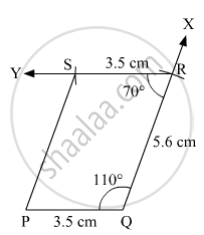SSC (English Medium) Class 8Maharashtra State Board
Share

# Construct ☐ Pqrs, Such that L(Pq) = 3.5 Cm, L(Qr) = 5.6 Cm, L(Rs) = 3.5 Cm, M∠Q = 110° , M∠R = 70° . If It is Given that ☐ Pqrs is a Parallelogram, Which of the Given Information is Unnecessary? - SSC (English Medium) Class 8 - Mathematics

ConceptKinds of Quadrilaterals Concept of Parallelogram

#### Question

Construct ☐ PQRS, such that l(PQ) = 3.5 cm, l(QR) = 5.6 cm, l(RS) = 3.5 cm, m∠Q = 110° , m∠R = 70° . If it is given that ☐ PQRS is a parallelogram, which of the given information is unnecessary?

#### Solution

Steps of Construction:

Step 1:
Draw PQ = 3.5 cm.

Step 2: Draw ∠PQX = 110° .

Step 3: With Q as centre and radius 5.6 cm, draw an arc cutting ray QX at R.

Step 4: Draw ∠QRY = 70° .

Step 5: With R as centre and radius 3.5 cm, draw an arc cutting ray RY at S.

Step 6: Join PS.Here, PQRS is the required quadrilateral.

If it is given that quadrilateral PQRS is a parallelogram, then the information l(RS) = 3.5 cm and m∠R = 70°  is unnecessary.

Is there an error in this question or solution?

#### APPEARS IN

Balbharati Solution for Balbharati Class 8 Mathematics (2019 to Current)
Chapter 8: Quadrilateral : Constructions and Types
Practice Set 8.3 | Q: 6 | Page no. 50
Solution Construct ☐ Pqrs, Such that L(Pq) = 3.5 Cm, L(Qr) = 5.6 Cm, L(Rs) = 3.5 Cm, M∠Q = 110° , M∠R = 70° . If It is Given that ☐ Pqrs is a Parallelogram, Which of the Given Information is Unnecessary? Concept: Kinds of Quadrilaterals - Concept of Parallelogram.
S